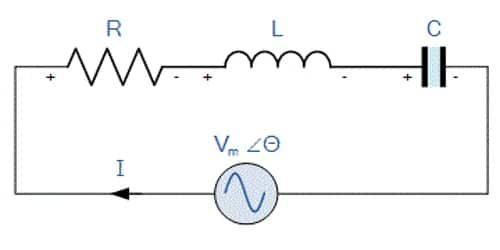# What is Acceptor Circuit?

The series resonant circuit is often called an ‘acceptor’ circuit. By offering minimum impedance to current at the resonant frequency it is able to select or accept most readily this particular frequency among many frequencies. Because at resonance, the impedance of the circuit is at its smallest so simply accept the current whose frequency is equivalent to its resonant frequency or we can say that it functions mostly at resonance.

The acceptor circuit provides the maximum response to currents at its resonant frequency. As a series resonance circuit, the only function on resonant frequency and the impedance of the acceptor circuit is minimum but the voltage can be magnified.

In radio receivers, the resonant frequency of the circuit is tuned to the frequency of the signal desired to be detected. This is usually done by varying the capacitance of a capacitor.

Characteristics of the series resonance circuit:

• Minimum impedance,
• Maximum circuit current,
• cos(φ) = 1, hence current and voltage becomes in phase,
• Circuit current becomes proportional to circuit resistance i.e. I ~ 1/R.

Uses of series resonance circuit:

• As frequency selection circuit in radio and TV tuner circuits.
• As a bandpass filter circuit.